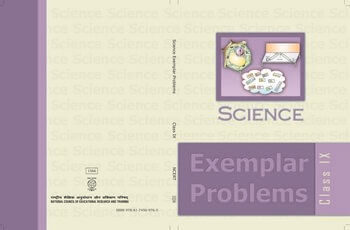•7. The work done on an object does not depend upon the (a) displacement (b) force applied (c) angle between force and displacement (d) initial velocity of the object 8. Water stored in a dam possesses (a) no energy (b) electrical energy (c) kinetic energy (d) potential energy h 9. A body is falling from a height h. After it has fallen a height , it will possess2 (a) only potential energy (b) only kinetic energy (c) half potential and half kinetic energy (d) more kinetic and less potential energy Short Answer Questions 10. A rocket is moving up with a velocityv. If the velocity of this rocket is suddenly tripled, what will be the ratio of two kinetic energies? 11. Avinash can run with a speed of 8 m s–1 against the frictional force of 10 N, and Kapil can move with a speed of 3 m s–1 against the frictional force of 25 N. Who is more powerful and why? 12. A boy is moving on a straight road against a frictional force of 5 N. After travelling a distance of 1.5 km he forgot the correct path at a round about (Fig. 11.1) of radius 100 m. However, he moves on the circular path for one and half cycle and then he moves forward upto 2.0 km. Calculate the work done by him. Fig. 11.1 13. Can any object have mechanical energy even if its momentum is zero? Explain. 14. Can any object have momentum even if its mechanical energy is zero? Explain. 15. The power of a motor pump is 2 kW. How much water per minute the pump can raise to a height of 10 m? (Given g = 10 m s–2) 16. The weight of a person on a planet A is about half that on the earth. He can jump upto 0.4 m height on the surface of the earth. How high he can jump on the planet A? WORK AND E NERGY